Anúncio

# Math project some applications of trigonometry

17 de Oct de 2014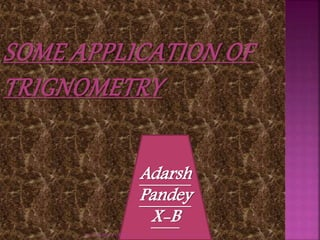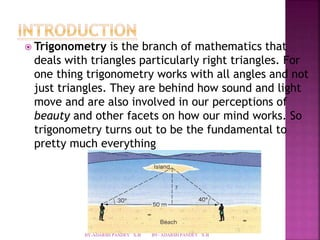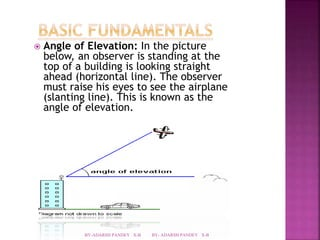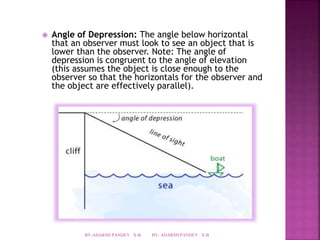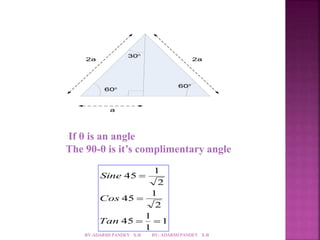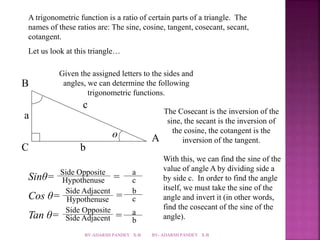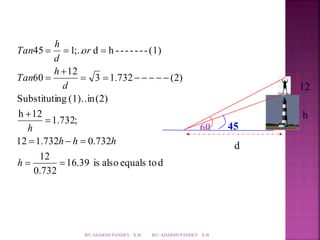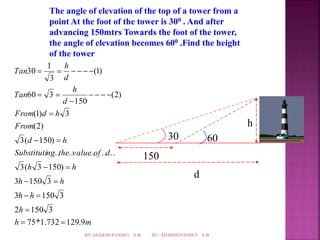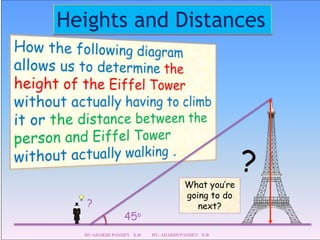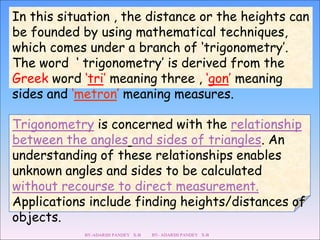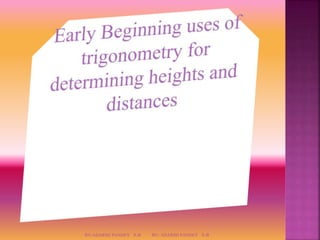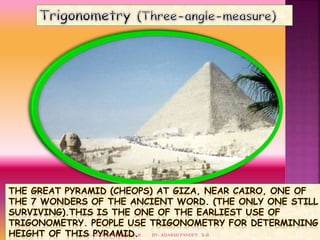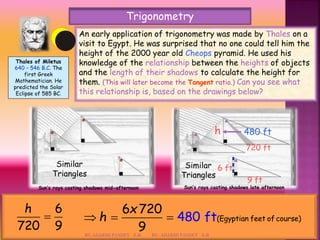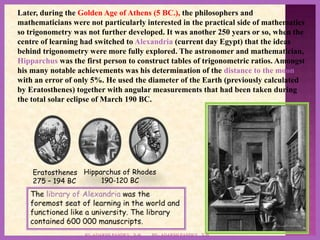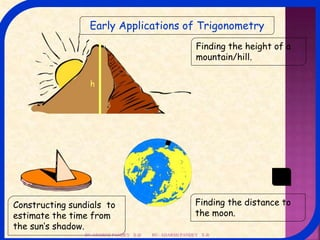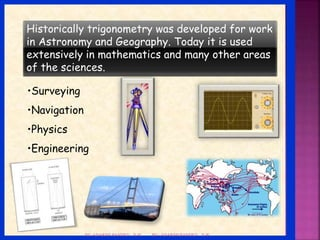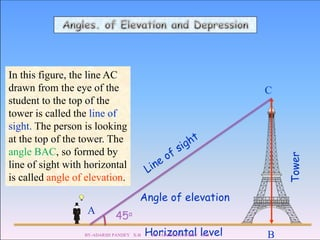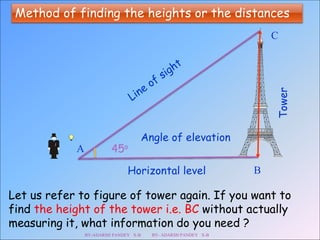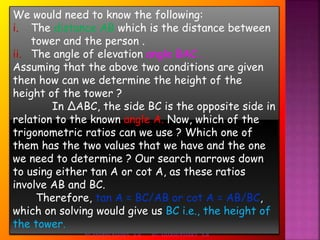1 de 21
Anúncio

### Math project some applications of trigonometry

2.  Trigonometry is the branch of mathematics that deals with triangles particularly right triangles. For one thing trigonometry works with all angles and not just triangles. They are behind how sound and light move and are also involved in our perceptions of beauty and other facets on how our mind works. So trigonometry turns out to be the fundamental to pretty much everything BY-ADARSH PANDEY X-B BY- ADARSH PANDEY X-B
3.  Angle of Elevation: In the picture below, an observer is standing at the top of a building is looking straight ahead (horizontal line). The observer must raise his eyes to see the airplane (slanting line). This is known as the angle of elevation. BY-ADARSH PANDEY X-B BY- ADARSH PANDEY X-B
4.  Angle of Depression: The angle below horizontal that an observer must look to see an object that is lower than the observer. Note: The angle of depression is congruent to the angle of elevation (this assumes the object is close enough to the observer so that the horizontals for the observer and the object are effectively parallel). BY-ADARSH PANDEY X-B BY- ADARSH PANDEY X-B
5. 2a 2a 600 300 600 a If θ is an angle The 90-θ is it’s complimentary angle 1 1 1  1 1 Sine 45 2 45 2 45    Cos Tan BY-ADARSH PANDEY X-B BY- ADARSH PANDEY X-B
6. A trigonometric function is a ratio of certain parts of a triangle. The names of these ratios are: The sine, cosine, tangent, cosecant, secant, cotangent. Let us look at this triangle… a c b ө A B C Given the assigned letters to the sides and angles, we can determine the following trigonometric functions. The Cosecant is the inversion of the sine, the secant is the inversion of the cosine, the cotangent is the inversion of the tangent. With this, we can find the sine of the value of angle A by dividing side a by side c. In order to find the angle itself, we must take the sine of the angle and invert it (in other words, find the cosecant of the sine of the angle). Sinθ= Cos θ= Tan θ= Side Opposite Hypothenuse Side Adjacent Hypothenuse Side Opposite Side Adjacent = = a c b c = a b BY-ADARSH PANDEY X-B BY- ADARSH PANDEY X-B
7. 60 45 12 h d h 45   1;.. d  h - - - - - - - (1) Substituting (1)..in (2) 1.732; h 12   12  1.732   0.732 16.39 is also equals to d 12 0.732 3 1.732 (2) 12 60            h h h h h d h Tan or d Tan BY-ADARSH PANDEY X-B BY- ADARSH PANDEY X-B
8. The angle of elevation of the top of a tower from a point At the foot of the tower is 300 . And after advancing 150mtrs Towards the foot of the tower, the angle of elevation becomes 600 .Find the height of the tower 150 h 30 60 d Tan Tan 1       From d h (1) 3 From h d d (2)  d h h 3( 150)   Substituting the value of d .. .. .. .. .. h h 3( 3 150)   h h 3 150 3   h h 3 150 3 h   2  150 3 h 75*1.732 129.9 m (2) 150 60 3 (1) 3 30          BY-ADARSH PANDEY X-B BY- ADARSH PANDEY X-B
9. Heights and Distances ? 45o ? What you’re going to do next? BY-ADARSH PANDEY X-B BY- ADARSH PANDEY X-B
10. In this situation , the distance or the heights can be founded by using mathematical techniques, which comes under a branch of ‘trigonometry’. The word ‘ trigonometry’ is derived from the Greek word ‘tri’ meaning three , ‘gon’ meaning sides and ‘metron’ meaning measures. Trigonometry is concerned with the relationship between the angles and sides of triangles. An understanding of these relationships enables unknown angles and sides to be calculated without recourse to direct measurement. Applications include finding heights/distances of objects. BY-ADARSH PANDEY X-B BY- ADARSH PANDEY X-B